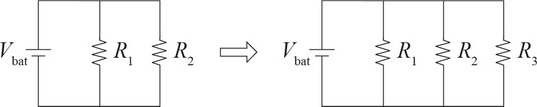# AP Physics 1 Question 415: Answer and Explanation

### Test Information

Question: 4153. Two resistors, R1 and R2, have equal resistance, and are connected in a circuit with a battery as shown in the figure above. If a third resistor, also with the same resistance, is then connected to the circuit, as shown, what happens to the energy E1 and E2 dissipated by R1 and R2, respectively, during a given time interval?

• A. E1 and E2 both decrease.
• B. E1 remains constant while E2 decreases.
• C. E1 decreases while E2 remains constant.
• D. E1 and E2 both remain constant.